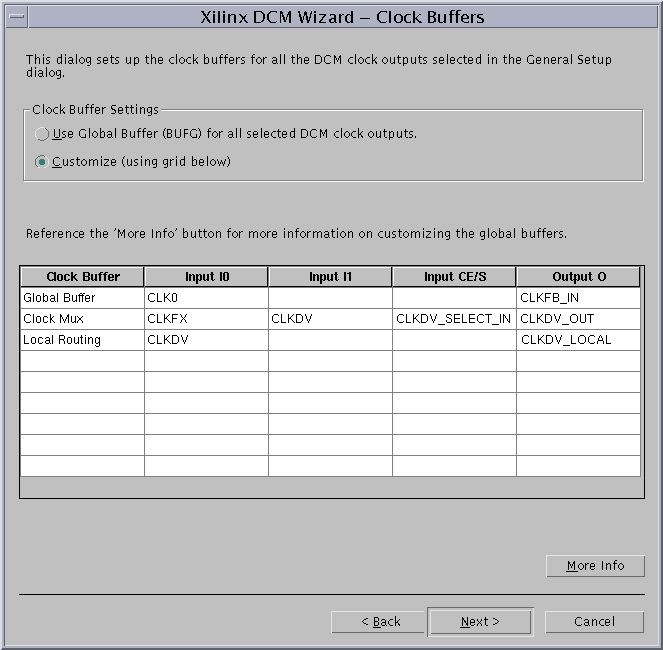# AR# 15468

|

## Description

General description:

The DCM Wizard (VHDL ONLY) writes incorrect VHDL code when a DCM output is connected to a clock buffer as well as a Local Routing/LOWSKEWLINE.

For example, if the CLKDV drives a BUFG and Local Routing (see image below), the DCM Wizard will use the port signal to make these connections. The resulting VHDL file will encounter an error during synthesis.CLKDV drives I1 of the BUFGMUX and "LOWSKEWLINE"

## Solution

This problem is fixed in the latest 5.1i Service Pack, available at:

http://support.xilinx.com/support/techsup/sw_updates
The first service pack containing the fix is 5.1i Service Pack 2.

Alternatively, you may also use the following work-around:

In this example VHDL, the four lines of code that must be updated are highlighted in bold text:

-- Module test_1

-- Generated by Xilinx Architecture Wizard

-- VHDL

-- Written for synthesis tool: XST

-- Xilinx Device: XC2V40-CS144-4

library IEEE;

use IEEE.std_logic_1164.all;

use IEEE.numeric_std.all;

-- synopsys translate_off

Library UNISIM;

use UNISIM.Vcomponents.all;

-- synopsys translate_on

entity test_1 is

port (

RST_IN : in std_logic;

CLKIN_IN : in std_logic;

CLKFX_SELECT_IN : in std_logic;

CLKDV_OUT : out std_logic;

LOCKED_OUT : out std_logic;

CLKFX_OUT : out std_logic;

CLK0_OUT : out std_logic);

attribute USELOWSKEWLINES : string;

attribute USELOWSKEWLINES of CLKDV_OUT : SIGNAL is "";

end test_1;

architecture STRUCT of test_1 is

signal CLKIN_IBUFG : std_logic;

signal CLKFB_IN : std_logic;

signal CLK0_BUF : std_logic;

signal CLKFX_BUF : std_logic;

signal GND : std_logic;

-- declare a new signal

signal CLKDV : std_logic;

attribute CLK_FEEDBACK : string;

attribute CLK_FEEDBACK of DCM_INST : label is "1X";

attribute CLKDV_DIVIDE : real;

attribute CLKDV_DIVIDE of DCM_INST : label is 2.0;

attribute CLKFX_DIVIDE : integer;

attribute CLKFX_DIVIDE of DCM_INST : label is 3;

attribute CLKFX_MULTIPLY : integer;

attribute CLKFX_MULTIPLY of DCM_INST : label is 4;

attribute CLKIN_DIVIDE_BY_2 : boolean;

attribute CLKIN_DIVIDE_BY_2 of DCM_INST : label is FALSE;

attribute CLKIN_PERIOD : real;

attribute CLKIN_PERIOD of DCM_INST : label is 10.0;

attribute CLKOUT_PHASE_SHIFT : string;

attribute CLKOUT_PHASE_SHIFT of DCM_INST : label is "NONE";

attribute DESKEW_ADJUST : string;

attribute DESKEW_ADJUST of DCM_INST : label is "SYSTEM_SYNCHRONOUS";

attribute DFS_FREQUENCY_MODE : string;

attribute DFS_FREQUENCY_MODE of DCM_INST : label is "LOW";

attribute DLL_FREQUENCY_MODE : string;

attribute DLL_FREQUENCY_MODE of DCM_INST : label is "LOW";

attribute DUTY_CYCLE_CORRECTION : boolean;

attribute DUTY_CYCLE_CORRECTION of DCM_INST : label is TRUE;

attribute PHASE_SHIFT : integer;

attribute PHASE_SHIFT of DCM_INST : label is 0;

attribute STARTUP_WAIT : boolean;

attribute STARTUP_WAIT of DCM_INST : label is FALSE;

-- Period Jitter (unit interval) = 0.09 UI

-- Period Jitter (Peak-to-Peak) = 0.68 ns

component DCM

-- synopsys translate_off

generic(

DFS_FREQUENCY_MODE : string := "LOW";

DLL_FREQUENCY_MODE : string := "LOW";

DUTY_CYCLE_CORRECTION : boolean := TRUE;

CLKIN_DIVIDE_BY_2 : boolean := FALSE;

CLK_FEEDBACK : string := "1X";

CLKOUT_PHASE_SHIFT : string := "NONE";

DSS_MODE : string := "NONE";

FACTORY_JF : bit_vector := X"C080";

STARTUP_WAIT : boolean := false;

PHASE_SHIFT : integer := 0;

CLKFX_MULTIPLY : integer := 4;

CLKFX_DIVIDE : integer := 1;

CLKDV_DIVIDE : real := 2.0;

CLKIN_PERIOD : real := 0.0;

DESKEW_ADJUST : string := "SYSTEM_SYNCHRONOUS"

);

-- synopsys translate_on

port (

CLKIN : in std_logic;

CLKFB : in std_logic;

RST : in std_logic;

PSEN : in std_logic;

PSINCDEC : in std_logic;

PSCLK : in std_logic;

DSSEN : in std_logic;

CLK0 : out std_logic;

CLK90 : out std_logic;

CLK180 : out std_logic;

CLK270 : out std_logic;

CLKDV : out std_logic;

CLK2X : out std_logic;

CLK2X180 : out std_logic;

CLKFX : out std_logic;

CLKFX180 : out std_logic;

STATUS : out std_logic_vector (7 downto 0);

LOCKED : out std_logic;

PSDONE : out std_logic

);

end component;

component IBUFG

port (

I : in std_logic;

O : out std_logic

);

end component;

component BUFG

port (

I : in std_logic;

O : out std_logic

);

end component;

component BUFGMUX

port (

I0 : in std_logic;

I1 : in std_logic;

S : in std_logic;

O : out std_logic

);

end component;

begin

DCM_INST : DCM

-- synopsys translate_off

Generic map (

CLK_FEEDBACK => "1X",

CLKDV_DIVIDE => 2.0,

CLKFX_DIVIDE => 3,

CLKFX_MULTIPLY => 4,

CLKIN_DIVIDE_BY_2 => FALSE,

CLKIN_PERIOD => 10.0,

CLKOUT_PHASE_SHIFT => "NONE",

DESKEW_ADJUST => "SYSTEM_SYNCHRONOUS",

DFS_FREQUENCY_MODE => "LOW",

DLL_FREQUENCY_MODE => "LOW",

DUTY_CYCLE_CORRECTION => TRUE,

PHASE_SHIFT => 0,

STARTUP_WAIT => FALSE)

-- synopsys translate_on

port map (

CLKIN => CLKIN_IBUFG,

CLKFB => CLKFB_IN,

RST => RST_IN,

PSEN => GND,

PSINCDEC => GND,

PSCLK => GND,

CLK0 => CLK0_BUF,

-- change the port map from 'CLKDV => CLKDV_OUT,' to

CLKDV => CLKDV,

CLKFX => CLKFX_BUF,

LOCKED => LOCKED_OUT);

CLKIN_IBUFG_INST : IBUFG

port map (

I => CLKIN_IN,

O => CLKIN_IBUFG);

CLK0_BUFG_INST : BUFG

port map (

I => CLK0_BUF,

O => CLKFB_IN);

CLKFX_BUFGMUX_INST : BUFGMUX

port map (

I0 => CLKFX_BUF,

-- change ' I1 => CLKDV_OUT, ' to

I1 => CLKDV,

S => CLKFX_SELECT_IN,

O => CLKFX_OUT);

CLK0_OUT <= CLKFB_IN;

GND <= '0';

-- Add a port assignment.

CLKDV_OUT <= CLKDV;

end STRUCT;

AR# 15468
Date 12/15/2012
Status Active
Type General Article
People Also Viewed